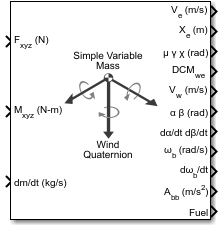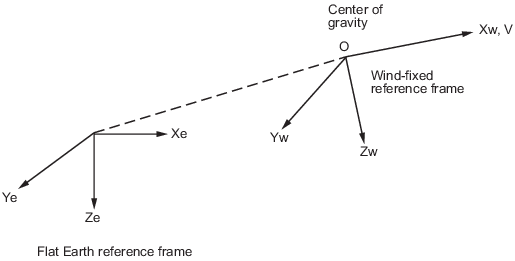Simple Variable Mass 6DOF Wind (Quaternion)

Implement quaternion representation of six-degrees-of-freedom equations of motion of simple variable mass with respect to wind axes

• Library:
• Aerospace Blockset / Equations of Motion / 6DOF

•Description

The Simple Variable Mass 6DOF Wind (Quaternion) block implements a quaternion representation of six-degrees-of-freedom equations of motion of simple variable mass with respect to wind axes. It considers the rotation of a wind-fixed coordinate frame (Xw, Yw, Zw) about an flat Earth reference frame (Xe, Ye, Ze).

Aerospace Blockset™ uses quaternions that are defined using the scalar-first convention. For more information on the wind-fixed coordinate frame, see Algorithms.

Limitations

The block assumes that the applied forces are acting at the center of gravity of the body.

Ports

Input

expand all

Applied forces, specified as a three-element vector.

Data Types: double

Applied moments, specified as a three-element vector.

Data Types: double

One or more rates of change of mass (positive if accreted, negative if ablated), specified as a scalar.

Data Types: double

One or more relative velocities, specified as a three-element vector, at which the mass is accreted to or ablated from the body in body-fixed axes.

Dependencies

To enable this port, select Include mass flow relative velocity.

Data Types: double

Output

expand all

Velocity in the flat Earth reference frame, returned as a three-element vector.

Data Types: double

Position in the flat Earth reference frame, returned as a three-element vector.

Data Types: double

Wind rotation angles [bank, flight path, heading], returned as three-element vector, in radians.

Data Types: double

Coordinate transformation from flat Earth axes to wind-fixed axes, returned as a 3-by-3 matrix.

Data Types: double

Velocity in wind-fixed frame, returned as a three-element vector.

Data Types: double

Angle of attack and sideslip angle, returned as a two-element vector, in radians.

Data Types: double

Rate of change of angle of attack and rate of change of sideslip angle, returned as a two-element vector, in radians per second.

Data Types: double

Angular rates in body-fixed axes, returned as a three-element vector.

Data Types: double

Angular accelerations in body-fixed axes, returned as a three-element vector, in radians per second squared.

Data Types: double

Accelerations in body-fixed axes with respect to body frame, returned as a three-element vector.

Data Types: double

Fuel tank status, returned as:

• 1 — Tank is full.

• 0 — Tank is neither full nor empty.

• -1 — Tank is empty.

Data Types: double

Accelerations in body-fixed axes with respect to inertial frame (flat Earth), returned as a three-element vector. You typically connect this signal to the accelerometer.

Dependencies

This port appears only when the Include inertial acceleration check box is selected.

Data Types: double

Parameters

expand all

Main

Input and output units, specified as Metric (MKS), English (Velocity in ft/s), or English (Velocity in kts).

UnitsForcesMomentAccelerationVelocityPositionMassInertia
Metric (MKS) NewtonNewton-meterMeters per second squaredMeters per secondMetersKilogramKilogram meter squared
English (Velocity in ft/s) PoundFoot-poundFeet per second squaredFeet per secondFeetSlugSlug foot squared
English (Velocity in kts) PoundFoot-poundFeet per second squaredKnotsFeetSlugSlug foot squared

Programmatic Use

 Block Parameter: units Type: character vector Values: Metric (MKS) | English (Velocity in ft/s) | English (Velocity in kts) Default: Metric (MKS)

Mass type, specified according to the following table.

Mass TypeDescriptionDefault For
Fixed

Mass is constant throughout the simulation.

Simple Variable

Mass and inertia vary linearly as a function of mass rate.

Custom Variable

Mass and inertia variations are customizable.

The Simple Variable selection conforms to the equations of motion in Algorithms.

Programmatic Use

 Block Parameter: mtype Type: character vector Values: Fixed | Simple Variable | Custom Variable Default: Simple Variable

Equations of motion representation, specified according to the following table.

RepresentationDescription

Quaternion

Use quaternions within equations of motion.

Wind Angles

Use wind angles within equations of motion.

The Quaternion selection conforms to the equations of motion in Algorithms.

Programmatic Use

 Block Parameter: rep Type: character vector Values: Wind Angles | Quaternion Default: 'Quaternion'

Initial location of the body in the flat Earth reference frame, specified as a three-element vector.

Programmatic Use

 Block Parameter: xme_0 Type: character vector Values: '[0 0 0]' | three-element vector Default: '[0 0 0]'

Initial airspeed, angle of attack, and sideslip angle, specified as a three-element vector.

Programmatic Use

 Block Parameter: Vm_0 Type: character vector Values: '[0 0 0]' | three-element vector Default: '[0 0 0]'

Initial wind angles [bank, flight path, and heading], specified as a three-element vector in radians.

Programmatic Use

 Block Parameter: wind_0 Type: character vector Values: '[0 0 0]' | three-element vector Default: '[0 0 0]'

Initial body-fixed angular rates with respect to the NED frame, specified as a three-element vector, in radians per second.

Programmatic Use

 Block Parameter: pm_0 Type: character vector Values: '[0 0 0]' | three-element vector Default: '[0 0 0]'

Initial mass of the rigid body, specified as a double scalar.

Programmatic Use

 Block Parameter: mass_0 Type: character vector Values: '1.0' | double scalar Default: '1.0'

Empty mass of the body, specified as a double scalar.

Programmatic Use

 Block Parameter: mass_e Type: character vector Values: double scalar Default: '0.5'

Full mass of the body, specified as a double scalar.

Programmatic Use

 Block Parameter: mass_f Type: character vector Values: double scalar Default: '2.0'

Inertia tensor matrix for the empty inertia of the body, specified as 3-by-3 matrix, in body-fixed axes.

Programmatic Use

 Block Parameter: inertia_e Type: character vector Values: 'eye(3)' | 3-by-3 matrix Default: 'eye(3)'

Inertia tensor matrix for the full inertia of the body, specified as a 3-by-3 matrix, in body-fixed axes.

Programmatic Use

 Block Parameter: inertia_f Type: character vector Values: '2*eye(3)' | 3-by-3 matrix Default: '2*eye(3)'

Select this check box to add a mass flow relative velocity port. This is the relative velocity at which the mass is accreted or ablated.

Programmatic Use

 Block Parameter: vre_flag Type: character vector Values: off | on Default: off

Select this check box to add an inertial acceleration port.

Dependencies

To enable the Abe port, select this parameter.

Programmatic Use

 Block Parameter: abi_flag Type: character vector Values: 'off' | 'on' Default: off

State Attributes

Assign a unique name to each state. You can use state names instead of block paths during linearization.

• To assign a name to a single state, enter a unique name between quotes, for example, 'velocity'.

• To assign names to multiple states, enter a comma-separated list surrounded by braces, for example, {'a', 'b', 'c'}. Each name must be unique.

• If a parameter is empty (' '), no name is assigned.

• The state names apply only to the selected block with the name parameter.

• The number of states must divide evenly among the number of state names.

• You can specify fewer names than states, but you cannot specify more names than states.

For example, you can specify two names in a system with four states. The first name applies to the first two states and the second name to the last two states.

• To assign state names with a variable in the MATLAB® workspace, enter the variable without quotes. A variable can be a character vector, cell array, or structure.

Position state names, specified as a comma-separated list surrounded by braces.

Programmatic Use

 Block Parameter: xme_statename Type: character vector Values: '' | comma-separated list surrounded by braces Default: ''

Velocity state names, specified as a character vector.

Programmatic Use

 Block Parameter: Vm_statename Type: character vector Values: '' | character vector Default: ''

Incidence angle state name, specified as a character vector.

Programmatic Use

 Block Parameter: alpha_statename Type: character vector Values: '' Default: ''

Sideslip angle state name, specified as a character vector.

Programmatic Use

 Block Parameter: beta_statename Type: character vector Values: '' Default: ''

Quaternion vector state names, specified as a comma-separated list surrounded by braces.

Programmatic Use

 Block Parameter: quat_statename Type: character vector Values: '' | comma-separated list surrounded by braces Default: ''

Body rotation rate state names, specified comma-separated list surrounded by braces.

Programmatic Use

 Block Parameter: pm_statename Type: character vector Values: '' | comma-separated list surrounded by braces Default: ''

Mass state name, specified as a character vector.

Programmatic Use

 Block Parameter: mass_statename Type: character vector Values: '' | character vector Default: ''

Algorithms

The origin of the wind-fixed coordinate frame is the center of gravity of the body, and the body is assumed to be rigid, an assumption that eliminates the need to consider the forces acting between individual elements of mass. The flat Earth reference frame is considered inertial, an excellent approximation that allows the forces due to the Earth's motion relative to the “fixed stars” to be neglected.The translational motion of the wind-fixed coordinate frame is given below, where the applied forces [Fx Fy Fz]T are in the wind-fixed frame. Vrew is the relative velocity in the wind axes at which the mass flow ($\stackrel{˙}{m}$) is ejected or added to the body.

$\begin{array}{l}{\overline{F}}_{w}=\left[\begin{array}{l}{F}_{x}\\ {F}_{y}\\ {F}_{z}\end{array}\right]=m\left({\stackrel{˙}{\overline{V}}}_{w}+{\overline{\omega }}_{w}×{\overline{V}}_{w}\right)+\stackrel{˙}{m}\overline{V}r{e}_{w}\\ {\overline{V}}_{w}=\left[\begin{array}{l}V\\ 0\\ 0\end{array}\right],{\overline{\omega }}_{w}=\left[\begin{array}{l}{p}_{w}\\ {q}_{w}\\ {r}_{w}\end{array}\right]=DM{C}_{wb}\left[\begin{array}{c}{p}_{b}-\stackrel{˙}{\beta }\mathrm{sin}\alpha \\ {q}_{b}-\stackrel{˙}{\alpha }\\ {r}_{b}+\stackrel{˙}{\beta }\mathrm{cos}\alpha \end{array}\right],{\overline{w}}_{b}\left[\begin{array}{l}{p}_{b}\\ {q}_{b}\\ {r}_{b}\end{array}\right]\end{array}$

The rotational dynamics of the body-fixed frame are given below, where the applied moments are [L MN]T, and the inertia tensor I is with respect to the origin O. Inertia tensor I is much easier to define in body-fixed frame.

$\begin{array}{l}{\overline{M}}_{b}=\left[\begin{array}{l}L\\ M\\ N\end{array}\right]=I{\stackrel{˙}{\overline{\omega }}}_{b}+{\overline{\omega }}_{b}×\left(I{\overline{\omega }}_{b}\right)+\stackrel{˙}{I}{\overline{\omega }}_{b}\\ I=\left[\begin{array}{lll}{I}_{xx}\hfill & -{I}_{xy}\hfill & -{I}_{xz}\hfill \\ -{I}_{yx}\hfill & {I}_{yy}\hfill & -{I}_{yz}\hfill \\ -{I}_{zx}\hfill & -{I}_{zy}\hfill & {I}_{zz}\hfill \end{array}\right]\end{array}$

The inertia tensor is determined using a table lookup which linearly interpolates between Ifull and Iempty based on mass (m). While the rate of change of the inertia tensor is estimated by the following equation.

$\stackrel{˙}{I}=\frac{{I}_{full}-{I}_{empty}}{{m}_{full}-{m}_{empty}}\stackrel{˙}{m}$

The integration of the rate of change of the quaternion vector is given below.

$\left[\begin{array}{l}{\stackrel{˙}{q}}_{0}\\ {\stackrel{˙}{q}}_{1}\\ {\stackrel{˙}{q}}_{2}\\ {\stackrel{˙}{q}}_{3}\end{array}\right]=-1/2\left[\begin{array}{llll}0\hfill & p\hfill & q\hfill & r\hfill \\ -p\hfill & 0\hfill & -r\hfill & q\hfill \\ -q\hfill & r\hfill & 0\hfill & -p\hfill \\ -r\hfill & -q\hfill & p\hfill & 0\hfill \end{array}\right]\left[\begin{array}{l}{q}_{0}\\ {q}_{1}\\ {q}_{2}\\ {q}_{3}\end{array}\right]$

 Stevens, Brian, and Frank Lewis. Aircraft Control and Simulation, 2nd ed. Hoboken, NJ: John Wiley & Sons, 2003.

 Zipfel, Peter H., Modeling and Simulation of Aerospace Vehicle Dynamics. 2nd ed. Reston, VA: AIAA Education Series, 2007.

Aerospace Blockset DocumentationGet trial now